# 你还弄不懂的傅里叶变换，神经网络只用了30多行代码就学会了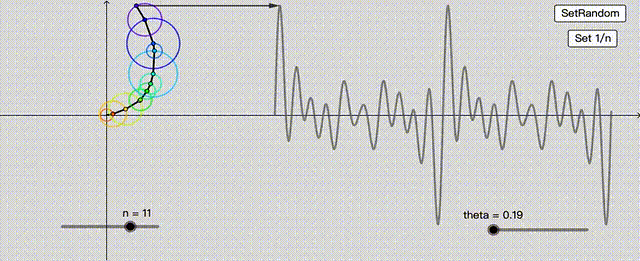# DFT=神经网络• k表示每N个样本的循环次数；
• N表示信号的长度；
• 表示信号在样本n处的值。

yk是一个复值，它给出了信号x中频率为k的正弦信号的信息；从yk我们可以计算正弦的振幅和相位。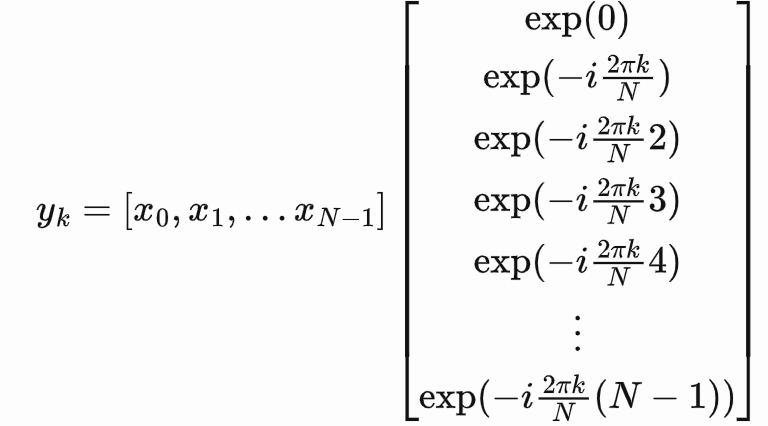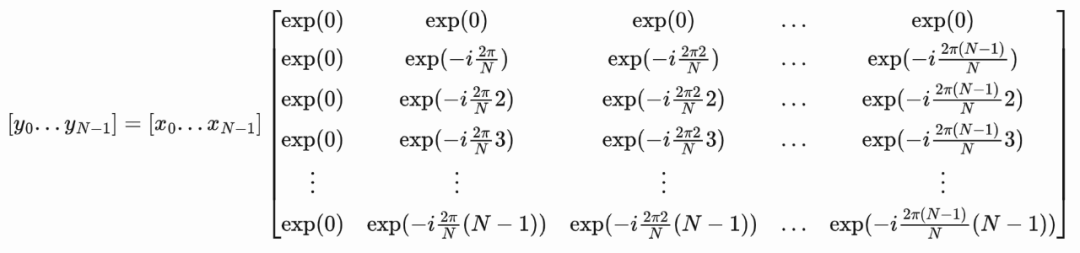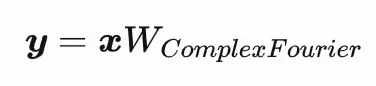• 不用复数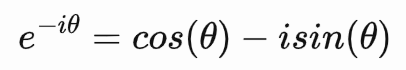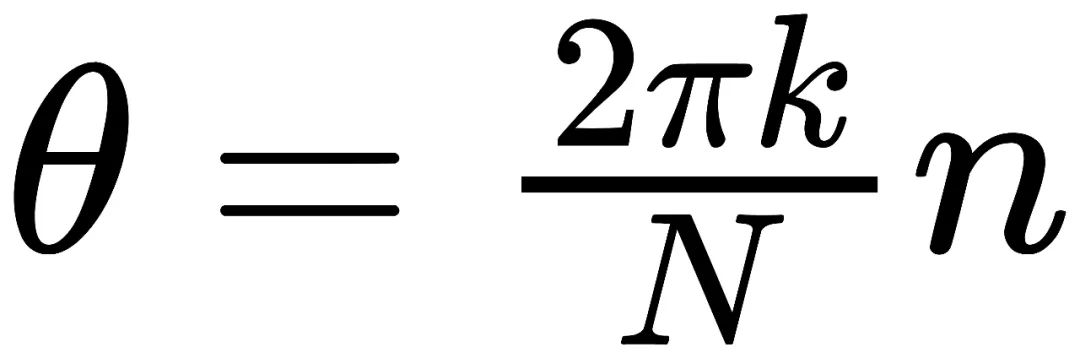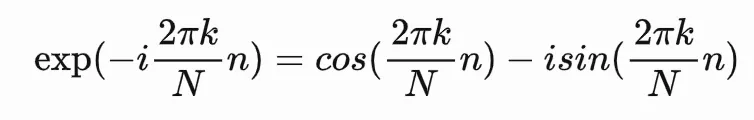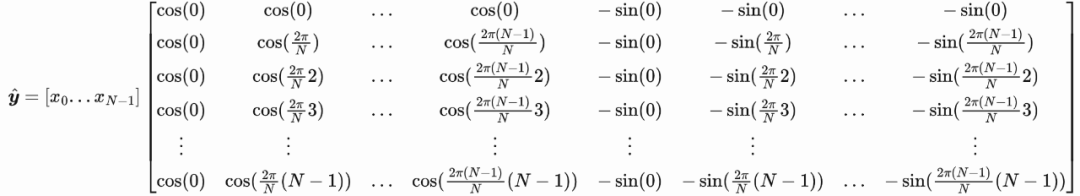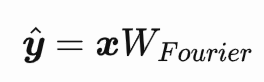# 用傅里叶权重计算傅里叶变换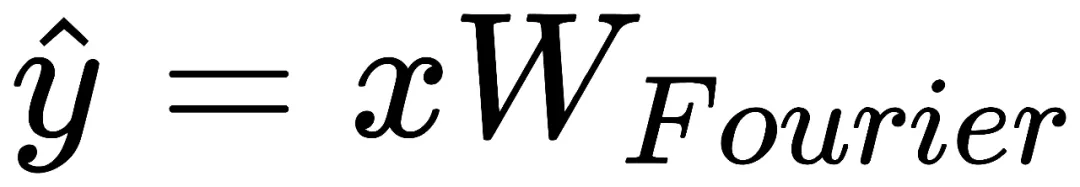，并用快速傅里叶变换（FFT）检查它是否正确。

``````import matplotlib.pyplot as plt

y_real = y[:, :signal_length]
y_imag = y[:, signal_length:]
tvals = np.arange(signal_length).reshape([-1, 1])
freqs = np.arange(signal_length).reshape([1, -1])
arg_vals = 2 * np.pi * tvals * freqs / signal_length
sinusoids = (y_real * np.cos(arg_vals) - y_imag * np.sin(arg_vals)) / signal_length
reconstructed_signal = np.sum(sinusoids, axis=1)

print('rmse:', np.sqrt(np.mean((x - reconstructed_signal)**2)))
plt.subplot(2, 1, 1)
plt.plot(x[0,:])
plt.title('Original signal')
plt.subplot(2, 1, 2)
plt.plot(reconstructed_signal)
plt.title('Signal reconstructed from sinusoids after DFT')
plt.tight_layout()
plt.show()``````
``rmse: 2.3243522568191728e-15``

• 另一种方法是重构信号
``````import matplotlib.pyplot as plt

y_real = y[:, :signal_length]
y_imag = y[:, signal_length:]
tvals = np.arange(signal_length).reshape([-1, 1])
freqs = np.arange(signal_length).reshape([1, -1])
arg_vals = 2 * np.pi * tvals * freqs / signal_length
sinusoids = (y_real * np.cos(arg_vals) - y_imag * np.sin(arg_vals)) / signal_length
reconstructed_signal = np.sum(sinusoids, axis=1)

print('rmse:', np.sqrt(np.mean((x - reconstructed_signal)**2)))
plt.subplot(2, 1, 1)
plt.plot(x[0,:])
plt.title('Original signal')
plt.subplot(2, 1, 2)
plt.plot(reconstructed_signal)
plt.title('Signal reconstructed from sinusoids after DFT')
plt.tight_layout()
plt.show()``````
``rmse: 2.3243522568191728e-15``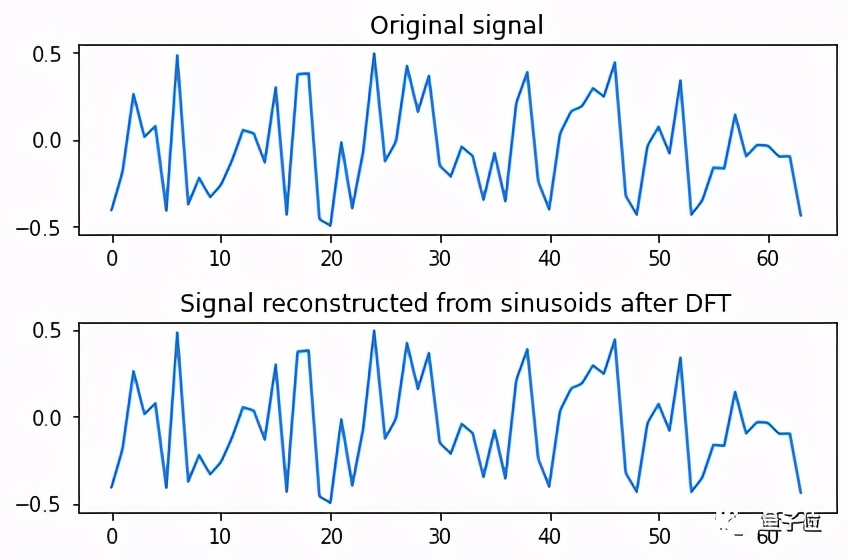# 通过梯度下降学习傅里叶变换

``````import tensorflow as tf

signal_length = 32

# Initialise weight vector to train:
W_learned = tf.Variable(np.random.random([signal_length, 2 * signal_length]) - 0.5)

# Expected weights, for comparison:
W_expected = create_fourier_weights(signal_length)

losses = []
rmses = []

for i in range(1000):
# Generate a random signal each iteration:
x = np.random.random([1, signal_length]) - 0.5

# Compute the expected result using the FFT:
fft = np.fft.fft(x)
y_true = np.hstack([fft.real, fft.imag])

y_pred = tf.matmul(x, W_learned)
loss = tf.reduce_sum(tf.square(y_pred - y_true))

# Train weights, via gradient descent:
W_learned = tf.Variable(W_learned - 0.1 * W_gradient)

losses.append(loss)
rmses.append(np.sqrt(np.mean((W_learned - W_expected)**2)))``````
``````Final loss value 1.6738563548424711e-09
Final weights' rmse value 3.1525832404710523e-06``````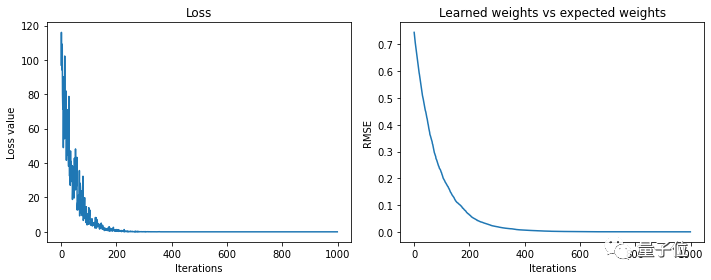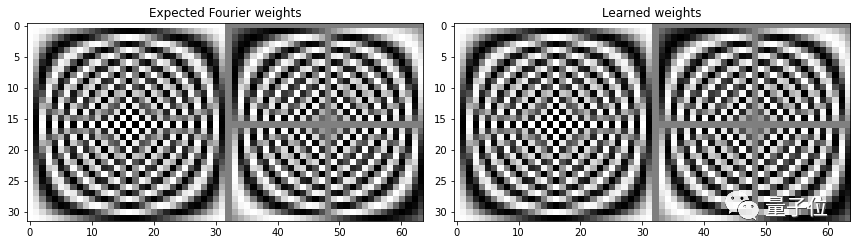# 训练网络学习DFT

``````W_learned = tf.Variable(np.random.random([signal_length, 2 * signal_length]) - 0.5)

tvals = np.arange(signal_length).reshape([-1, 1])
freqs = np.arange(signal_length).reshape([1, -1])
arg_vals = 2 * np.pi * tvals * freqs / signal_length
cos_vals = tf.cos(arg_vals) / signal_length
sin_vals = tf.sin(arg_vals) / signal_length

losses = []
rmses = []

for i in range(10000):
x = np.random.random([1, signal_length]) - 0.5

y_pred = tf.matmul(x, W_learned)
y_real = y_pred[:, 0:signal_length]
y_imag = y_pred[:, signal_length:]
sinusoids = y_real * cos_vals - y_imag * sin_vals
reconstructed_signal = tf.reduce_sum(sinusoids, axis=1)
loss = tf.reduce_sum(tf.square(x - reconstructed_signal))

W_learned = tf.Variable(W_learned - 0.5 * W_gradient)

losses.append(loss)
rmses.append(np.sqrt(np.mean((W_learned - W_expected)**2)))``````
``````Final loss value 4.161919455121241e-22
Final weights' rmse value 0.20243339269590094``````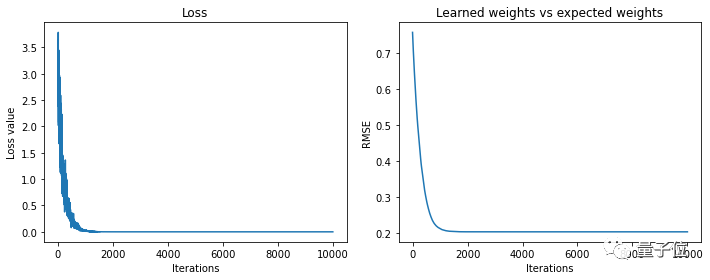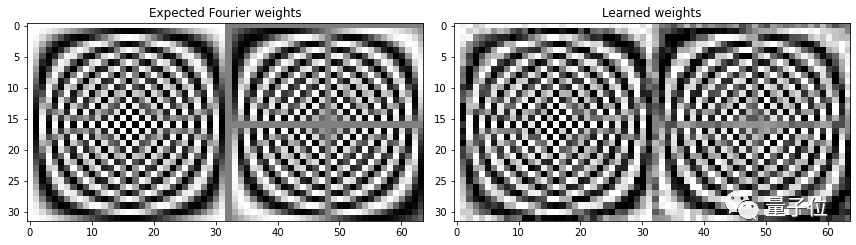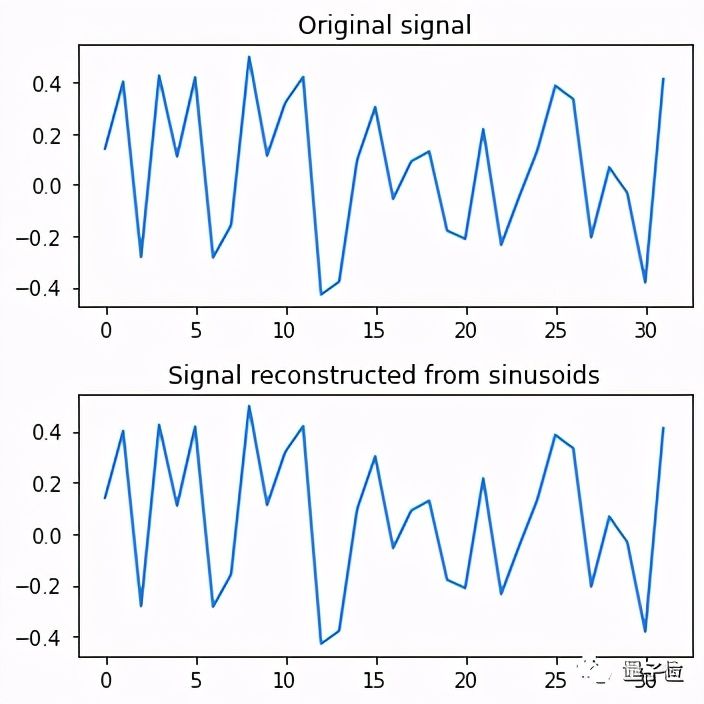``````W_learned = tf.Variable(np.random.random([signal_length, 2 * signal_length]) - 0.5)

losses = []
rmses = []

for i in range(10000):
x = np.random.random([1, signal_length]) - .5

y_pred = tf.matmul(x, W_learned)
y_real = y_pred[:, 0:signal_length]
y_imag = y_pred[:, signal_length:]
amplitudes = tf.sqrt(y_real**2 + y_imag**2) / signal_length
phases = tf.atan2(y_imag, y_real)
sinusoids = amplitudes * tf.cos(arg_vals + phases)
reconstructed_signal = tf.reduce_sum(sinusoids, axis=1)
loss = tf.reduce_sum(tf.square(x - reconstructed_signal))

W_learned = tf.Variable(W_learned - 0.5 * W_gradient)

losses.append(loss)
rmses.append(np.sqrt(np.mean((W_learned - W_expected)**2)))``````
``````Final loss value 2.2379359316633115e-21
Final weights' rmse value 0.2080118219691059``````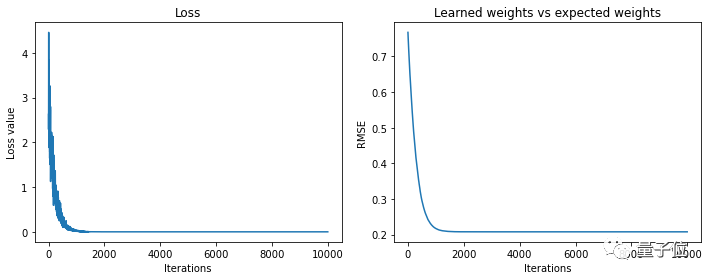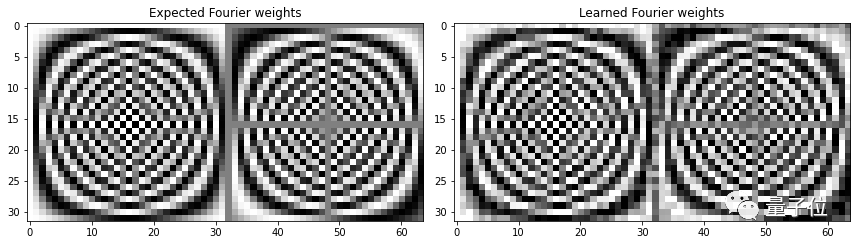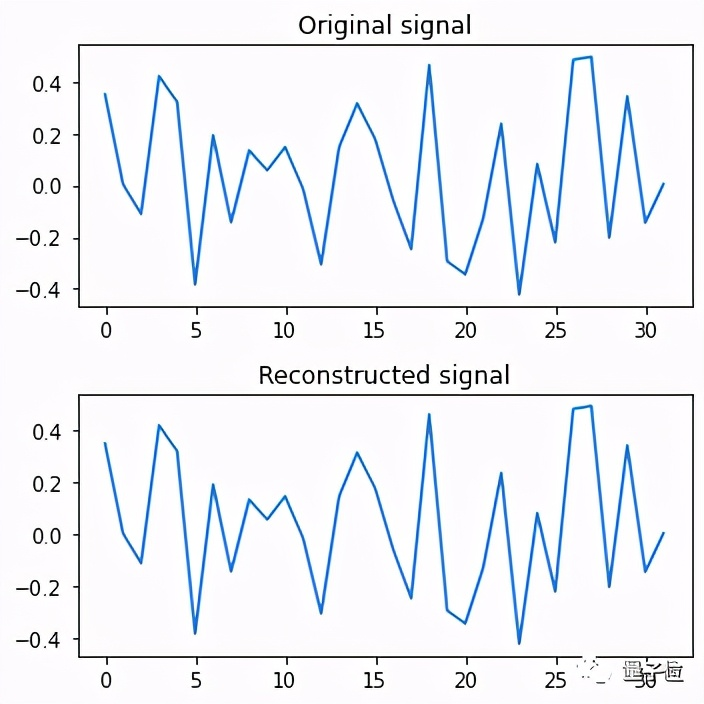• 值得一提的是，这个方法目前还有疑问存在：

https://sidsite.com/posts/fourier-nets/